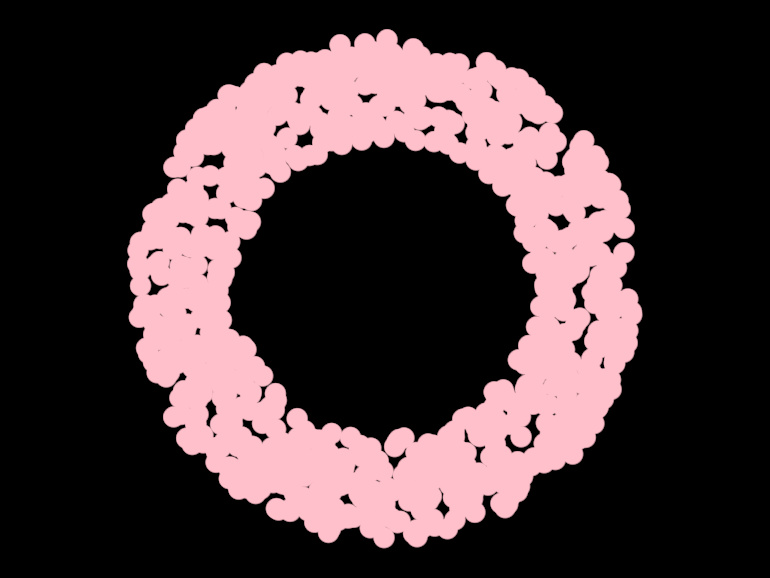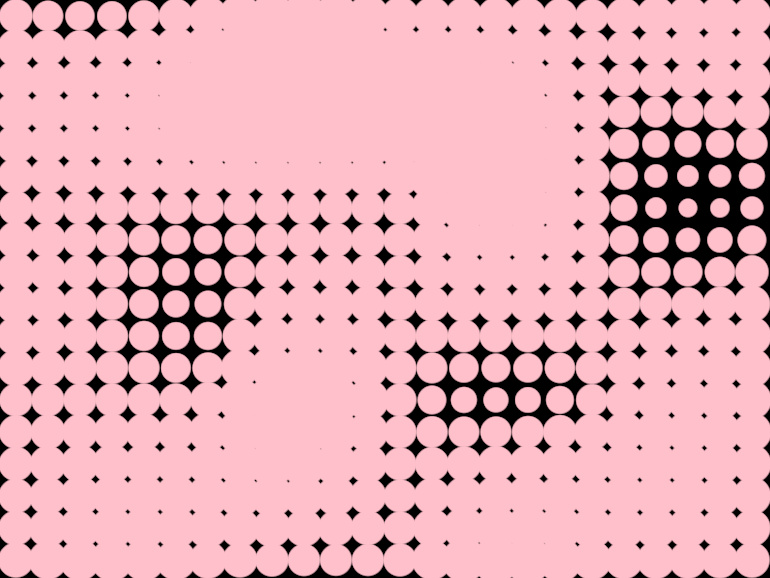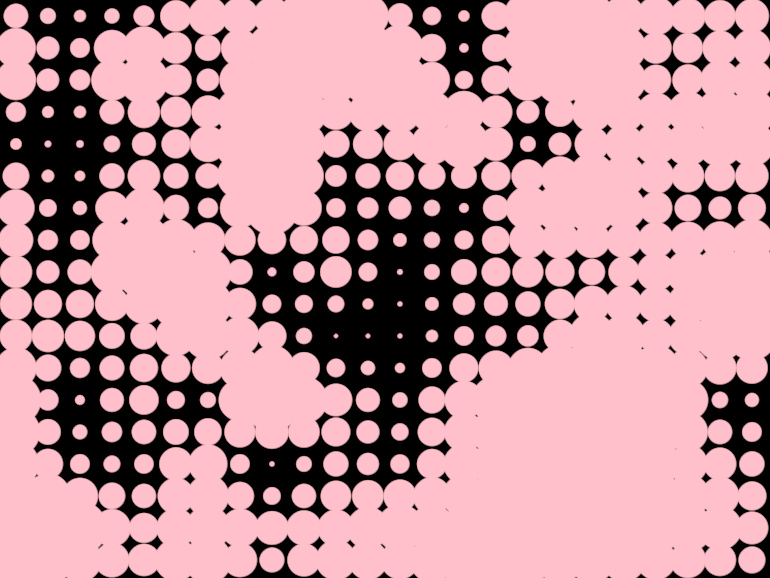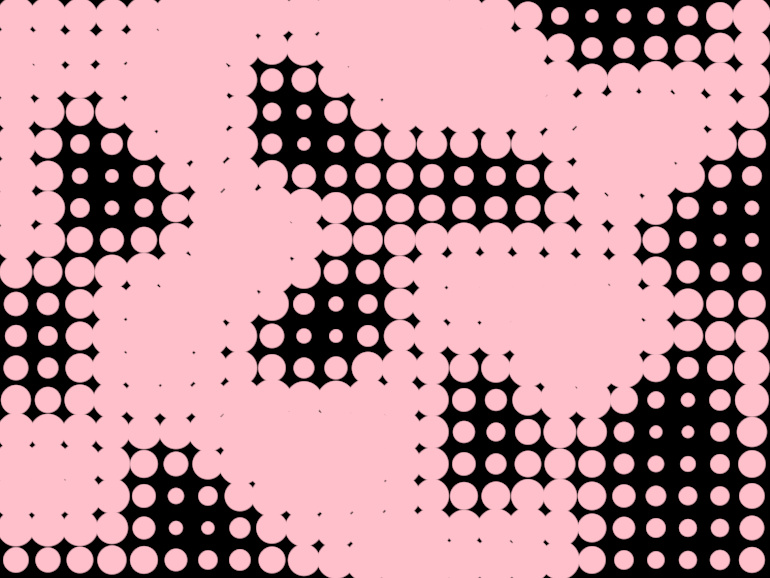# orx-noise

A collection of noise generator functions. Source and extra documentation can be found in the orx-noise sourcetree.

## Prerequisites

Assuming you are working on an `openrndr-template` based project, all you have to do is enable `orx-noise` in the `orxFeatures` set in `build.gradle.kts` and reimport the gradle project.

## Uniformly distributed random values

The library provides extension methods for `Double`, `Vector2`, `Vector3`, `Vector4` to create random vectors easily. To create scalars and vectors with uniformly distributed noise you use the `uniform` extension function.

``````val d1 = Double.uniform(0.0, 640.0)
val v2 = Vector2.uniform(0.0, 640.0)
val v3 = Vector3.uniform(0.0, 640.0)
val v4 = Vector4.uniform(0.0, 640.0)
``````

To create multiple samples of noise one uses the `uniforms` function.

``````val v2 = Vector2.uniforms(100, Vector2(0.0, 0.0), Vector2(640.0, 640.0))
val v3 = Vector3.uniforms(100, Vector3(0.0, 0.0, 0.0), Vector3(640.0, 640.0, 640.0))
``````

The `Random` class can also be used to generate Double numbers and vector, but also booleans and integers.

``````// Boolean
val b = Random.bool(probability = 0.2)

// Int
val i1 = Random.int(0, 640)
val i2 = Random.int0(640)

// Double
val d2 = Random.double(0.0, 640.0)
val d3 = Random.double0(640.0)

// Vectors
val v2 = Random.vector2(0.0, 640.0)
val v3 = Random.vector3(0.0, 640.0)
val v4 = Random.vector4(0.0, 640.0)
``````

## Perlin, Value and Simplex noise

`Random.perlin()` and `Random.value()` accept 2D and 3D arguments. `Random.simplex()` up to 4D. They all return a `Double`. Some examples:

``````// Test vectors to use
val v2 = Vector2(0.1, 0.2)
val v4 = Vector4(0.1, 0.2, 0.3, 0.4)

// Now generate random values
val d1 = Random.perlin(0.1, 0.2)
val d2 = Random.perlin(v2)
val d3 = Random.value(0.1, 0.2, 0.3)
val d4 = Random.simplex(v4)
``````

## Uniform ring noise

``````val v2 = Vector2.uniformRing(0.0, 300.0)
val v3 = Vector3.uniformRing(0.0, 300.0)
val v4 = Vector4.uniformRing(0.0, 300.0)
````````````fun main() = application {
program {
extend {
drawer.fill = ColorRGBa.PINK
drawer.stroke = null
drawer.translate(width / 2.0, height / 2.00)
for (i in 0 until 1000) {
drawer.circle(Vector2.uniformRing(150.0, 250.0), 10.0)
}
}
}
}
``````

## Perlin noise``````fun main() = application {
program {
extend {
drawer.fill = ColorRGBa.PINK
drawer.stroke = null
val scale = 0.005
for (y in 16 until height step 32) {
for (x in 16 until width step 32) {
val radius = perlinLinear(100, x * scale, y * scale) * 16.0 + 16.0
drawer.circle(x * 1.0, y * 1.0, radius)
}
}
}
}
}
``````

## Value noise``````fun main() = application {
program {
extend {
drawer.fill = ColorRGBa.PINK
drawer.stroke = null
val scale = 0.0150
for (y in 16 until height step 32) {
for (x in 16 until width step 32) {
val radius = valueLinear(100, x * scale, y * scale) * 16.0 + 16.0
drawer.circle(x * 1.0, y * 1.0, radius)
}
}
}
}
}
``````

## Simplex noise``````fun main() = application {
program {
extend {
drawer.fill = ColorRGBa.PINK
drawer.stroke = null
val scale = 0.004
for (y in 16 until height step 32) {
for (x in 16 until width step 32) {
val radius = simplex(100, x * scale, y * scale) * 16.0 + 16.0
drawer.circle(x * 1.0, y * 1.0, radius)
}
}
}
}
}
``````

## Fractal/FBM noise

``````fun main() = application {
program {
extend {
drawer.fill = ColorRGBa.PINK
drawer.stroke = null
val s = 0.0080
val t = seconds
for (y in 4 until height step 8) {
for (x in 4 until width step 8) {
t < 3.0 -> abs(fbm(100, x * s, y * s, t, ::perlinLinear)) * 16.0
t < 6.0 -> billow(100, x * s, y * s, t, ::perlinLinear) * 2.0
else -> rigid(100, x * s, y * s, t, ::perlinLinear) * 16.0
}
drawer.circle(x * 1.0, y * 1.0, radius)
}
}
}
}
}
``````

Noise functions have evaluable gradients, a direction to where the value of the function increases the fastest. The `gradient1D`, `gradient2D`, `gradient3D` and `gradient4D` functions can be used to estimate gradients for noise functions.

``````fun main() = application {
program {
extend {
drawer.fill = null
drawer.stroke = ColorRGBa.PINK
drawer.lineCap = LineCap.ROUND
drawer.strokeWeight = 3.0
val t = seconds
for (y in 4 until height step 8) {
for (x in 4 until width step 8) {
val g = gradient3D(::perlinQuintic, 100, x * 0.005, y * 0.005, t, 0.0005).xy
drawer.lineSegment(Vector2(x * 1.0, y * 1.0) - g * 2.0, Vector2(x * 1.0, y * 1.0) + g * 2.0)
}
}
}
}
}
``````

Gradients can also be calculated for the fbm, rigid and billow versions of the noise functions. However, we first have to create a function that can be used by the gradient estimator. For this `fbmFunc3D`, `billowFunc3D`, and `rigidFunc3D` can be used (which works through partial application).

``````fun main() = application {
program {
val noise = fbmFunc3D(::simplex, octaves = 3)
extend {
drawer.fill = null
drawer.stroke = ColorRGBa.PINK
drawer.lineCap = LineCap.ROUND
drawer.strokeWeight = 1.5
val t = seconds
for (y in 4 until height step 8) {
for (x in 4 until width step 8) {
val g = gradient3D(noise, 100, x * 0.002, y * 0.002, t, 0.002).xy
drawer.lineSegment(Vector2(x * 1.0, y * 1.0) - g * 1.0, Vector2(x * 1.0, y * 1.0) + g * 1.0)
}
}
}
}
}
``````